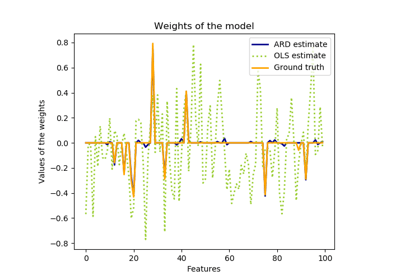/scikit-learn

# sklearn.linear_model.ARDRegression

`class sklearn.linear_model.ARDRegression(n_iter=300, tol=0.001, alpha_1=1e-06, alpha_2=1e-06, lambda_1=1e-06, lambda_2=1e-06, compute_score=False, threshold_lambda=10000.0, fit_intercept=True, normalize=False, copy_X=True, verbose=False)` [source]

Bayesian ARD regression.

Fit the weights of a regression model, using an ARD prior. The weights of the regression model are assumed to be in Gaussian distributions. Also estimate the parameters lambda (precisions of the distributions of the weights) and alpha (precision of the distribution of the noise). The estimation is done by an iterative procedures (Evidence Maximization)

Read more in the User Guide.

Parameters: `n_iter : int, optional` Maximum number of iterations. Default is 300 `tol : float, optional` Stop the algorithm if w has converged. Default is 1.e-3. `alpha_1 : float, optional` Hyper-parameter : shape parameter for the Gamma distribution prior over the alpha parameter. Default is 1.e-6. `alpha_2 : float, optional` Hyper-parameter : inverse scale parameter (rate parameter) for the Gamma distribution prior over the alpha parameter. Default is 1.e-6. `lambda_1 : float, optional` Hyper-parameter : shape parameter for the Gamma distribution prior over the lambda parameter. Default is 1.e-6. `lambda_2 : float, optional` Hyper-parameter : inverse scale parameter (rate parameter) for the Gamma distribution prior over the lambda parameter. Default is 1.e-6. `compute_score : boolean, optional` If True, compute the objective function at each step of the model. Default is False. `threshold_lambda : float, optional` threshold for removing (pruning) weights with high precision from the computation. Default is 1.e+4. `fit_intercept : boolean, optional` whether to calculate the intercept for this model. If set to false, no intercept will be used in calculations (e.g. data is expected to be already centered). Default is True. `normalize : boolean, optional, default False` This parameter is ignored when `fit_intercept` is set to False. If True, the regressors X will be normalized before regression by subtracting the mean and dividing by the l2-norm. If you wish to standardize, please use `sklearn.preprocessing.StandardScaler` before calling `fit` on an estimator with `normalize=False`. `copy_X : boolean, optional, default True.` If True, X will be copied; else, it may be overwritten. `verbose : boolean, optional, default False` Verbose mode when fitting the model. `coef_ : array, shape = (n_features)` Coefficients of the regression model (mean of distribution) `alpha_ : float` estimated precision of the noise. `lambda_ : array, shape = (n_features)` estimated precisions of the weights. `sigma_ : array, shape = (n_features, n_features)` estimated variance-covariance matrix of the weights `scores_ : float` if computed, value of the objective function (to be maximized)

#### Notes

For an example, see examples/linear_model/plot_ard.py.

#### References

D. J. C. MacKay, Bayesian nonlinear modeling for the prediction competition, ASHRAE Transactions, 1994.

R. Salakhutdinov, Lecture notes on Statistical Machine Learning, http://www.utstat.toronto.edu/~rsalakhu/sta4273/notes/Lecture2.pdf#page=15 Their beta is our `self.alpha_` Their alpha is our `self.lambda_` ARD is a little different than the slide: only dimensions/features for which `self.lambda_ < self.threshold_lambda` are kept and the rest are discarded.

#### Examples

```>>> from sklearn import linear_model
>>> clf = linear_model.ARDRegression()
>>> clf.fit([[0,0], [1, 1], [2, 2]], [0, 1, 2])
...
ARDRegression(alpha_1=1e-06, alpha_2=1e-06, compute_score=False,
copy_X=True, fit_intercept=True, lambda_1=1e-06, lambda_2=1e-06,
n_iter=300, normalize=False, threshold_lambda=10000.0, tol=0.001,
verbose=False)
>>> clf.predict([[1, 1]])
array([1.])
```

#### Methods

 `fit`(X, y) Fit the ARDRegression model according to the given training data and parameters. `get_params`([deep]) Get parameters for this estimator. `predict`(X[, return_std]) Predict using the linear model. `score`(X, y[, sample_weight]) Returns the coefficient of determination R^2 of the prediction. `set_params`(**params) Set the parameters of this estimator.
`__init__(n_iter=300, tol=0.001, alpha_1=1e-06, alpha_2=1e-06, lambda_1=1e-06, lambda_2=1e-06, compute_score=False, threshold_lambda=10000.0, fit_intercept=True, normalize=False, copy_X=True, verbose=False)` [source]
`fit(X, y)` [source]

Fit the ARDRegression model according to the given training data and parameters.

Iterative procedure to maximize the evidence

Parameters: `X : array-like, shape = [n_samples, n_features]` Training vector, where n_samples in the number of samples and n_features is the number of features. `y : array, shape = [n_samples]` Target values (integers). Will be cast to X’s dtype if necessary `self : returns an instance of self.`
`get_params(deep=True)` [source]

Get parameters for this estimator.

Parameters: `deep : boolean, optional` If True, will return the parameters for this estimator and contained subobjects that are estimators. `params : mapping of string to any` Parameter names mapped to their values.
`predict(X, return_std=False)` [source]

Predict using the linear model.

In addition to the mean of the predictive distribution, also its standard deviation can be returned.

Parameters: `X : {array-like, sparse matrix}, shape = (n_samples, n_features)` Samples. `return_std : boolean, optional` Whether to return the standard deviation of posterior prediction. `y_mean : array, shape = (n_samples,)` Mean of predictive distribution of query points. `y_std : array, shape = (n_samples,)` Standard deviation of predictive distribution of query points.
`score(X, y, sample_weight=None)` [source]

Returns the coefficient of determination R^2 of the prediction.

The coefficient R^2 is defined as (1 - u/v), where u is the residual sum of squares ((y_true - y_pred) ** 2).sum() and v is the total sum of squares ((y_true - y_true.mean()) ** 2).sum(). The best possible score is 1.0 and it can be negative (because the model can be arbitrarily worse). A constant model that always predicts the expected value of y, disregarding the input features, would get a R^2 score of 0.0.

Parameters: `X : array-like, shape = (n_samples, n_features)` Test samples. For some estimators this may be a precomputed kernel matrix instead, shape = (n_samples, n_samples_fitted], where n_samples_fitted is the number of samples used in the fitting for the estimator. `y : array-like, shape = (n_samples) or (n_samples, n_outputs)` True values for X. `sample_weight : array-like, shape = [n_samples], optional` Sample weights. `score : float` R^2 of self.predict(X) wrt. y.
`set_params(**params)` [source]

Set the parameters of this estimator.

The method works on simple estimators as well as on nested objects (such as pipelines). The latter have parameters of the form `<component>__<parameter>` so that it’s possible to update each component of a nested object.

Returns: self

## Examples using `sklearn.linear_model.ARDRegression`Automatic Relevance Determination Regression (ARD)